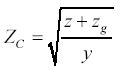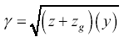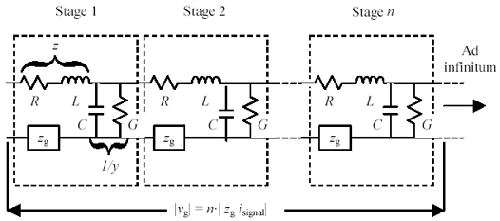# Impedance in Series with the Return Path

A single-ended transmission structure (as opposed to a differential transmission structure) comprises two conductors ”one for the signal current and one for the returning signal current. These conductors are called the signal conductor and the return conductor respectively. High-speed digital pcbs use a solid reference plane for the return conductor. Although many digital engineers focus their attention on the signal conductor, both conductors play equally important roles in the transmission of high-speed signals.

Consider a transmission line with series impedance and shunt conductance z and y respectively per unit length (Figure 2.31), modeled as shown by per-unit-length parameters R , L , G , and C . To the return conductor of that structure add an impedance z g per unit length. The values of input impedance Z C and transmission coefficient g are modified to become

Equation 2.104Equation 2.105Figure 2.31. Any impedance placed in series with the return path increases Z , increases g , and induces a ground shift voltage v gAs indicated by equations [2.104] and [2.105], the impedance z g effectively adds to the total per-unit-length series impedance of the structure. Impedance z g increases the apparent input impedance of the structure and also increases the high-frequency loss.

A lumped-element series-connected discontinuity inserted into a transmission structure causes equal amounts of disruption whether it is inserted in series with the signal path or the return path.

In high-speed pcb problems the return-path impedance comprises both the resistance and inductance of the solid reference planes. The resistive component of the return-path impedance would be negligible if the returning signal current were allowed to spread perfectly across the entire reference plane; however, that doesn't happen. At high frequencies the returning signal current bunches together, flowing only in a narrow band directly underneath the signal trace on the nearest reference plane. In a typical 50-ohm microstrip or stripline at height h above the nearest solid reference plane, 80% of the returning signal current in the reference plane flows within about 3 h on either side of the signal trace. The reference-plane resistance adds to the total power dissipation of the structure, increasing the signal attenuation (see Section 2.10.3, "Proximity Effect for Microstrip and Stripline Circuits"). The skin-effect loss calculations presented in Chapter 5 take into account the location and profile of current on the reference plane. Such calculations are a normal part of any 2-D field solver that reports skin-effect loss.

What is not properly taken into account in most 2-D field solvers is the inductance of the return pathway . Most field solvers assume the reference plane is either infinite in extent (using the image-plane method) or a closed surface wrapped around the trace at a safe distance (using finite-element simulation of the wrapped reference plane). Either approach produces near-correct values for the overall per-unit-length inductance of the structure. What these approaches do not do is apportion that inductance into that part which appears in series with the signal conductor and that part in series with the ground. While the apportionment of inductance does not affect the line impedance or the transmission coefficient, it does affect the ground voltages measured from end to end across the structure, which in turn has a major effect on electromagnetic radiation and susceptibility.

When working with differential pairs, this discussion should remind you to add the series resistance of both wires when calculating the system loss. In working with coaxial cables, you must sum the resistance of the inner conductor and the resistance of the shield to find the total effective series resistance.

POINT TO REMEMBER

• An impedance in series with the return path affects the signal just as much as an impedance in series with the signal conductor.High-Speed Signal Propagation[c] Advanced Black Magic
ISBN: 013084408X
EAN: N/A
Year: 2005
Pages: 163